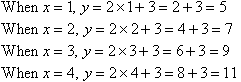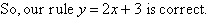Year 7 Interactive Maths - Second Edition

Modelling Linear Relationships

Tables and graphs are useful to find a pattern between the y-coordinate and the x-coordinate.

Using Tables

We can find a pattern in coordinates by listing their ordered pairs in a table.

Example 7

Consider the following table.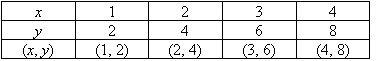a.  Describe the relationship between the y-coordinate and the x-coordinate in words.
b.  Find the algebraic relationship between x and y.

Solution:

a.  By trial and error, we look for a relationship between the values of x and y.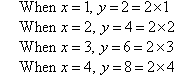We can describe the relationship between x and y in words as follows:
The y-coordinate is twice the x-coordinate
This means y is twice x.

b.  So, the algebraic relationship between the x-coordinate and y-coordinate is: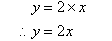Using Graphs

We can find a pattern in coordinates by drawing a graph of their ordered pairs.

Example 8

a.  Plot the following points on a Cartesian plane:
A(1, 3), B(2, 6), C(3, 9), D(4, 12), E(5, 15), F(6, 18)
Use a ruler to join the points A, B, C, D, E and F.

b.  Describe the relationship between the y-coordinate and the x-coordinate in words.
c.  Find the algebraic relationship between x and y.

Solution: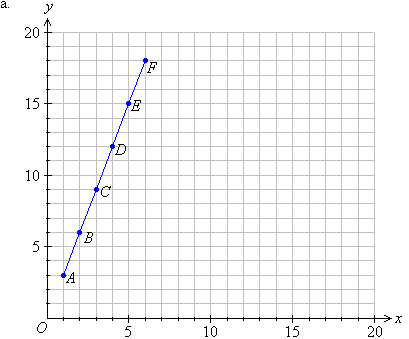b.  We notice that the points lie on a line.  Such a pattern is called a linear relationship because it represents a straight line relationship between the coordinates of the points.

We can describe the relationship between x and y in words as follows:  The y-coordinate is three times the x-coordinate.  This means y is three times x.

c.  So, the algebraic relationship between the x-coordinate and y-coordinate is: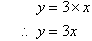Example 9

a.  Plot the following points on a Cartesian plane:
A(2, 0), B(3, 1), C(4, 2), D(5, 3), E(6, 4) and F(7, 5)
Use a ruler to join the points A, B, C, D, E and F.

b.  Describe the relationship between the y-coordinate and the x-coordinate in words.
c.  Find the algebraic relationship between x and y.

Solution: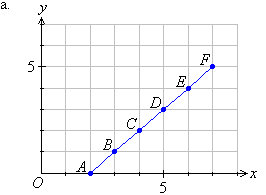b.  We notice that the points lie on a line.  Such a pattern is called a linear relationship because it represents a straight line relationship between the coordinates of the points in the pattern.

We can describe the relationship between x and y in words as follows:
The y-coordinate is two less than the x-coordinate

c.  So algebraically, the relationship between the x-coordinate and y-coordinate is: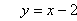Using a Difference Pattern

When we look for a pattern in ordered pairs, we can find the difference between two successive values of y.  This allows us to find a rule as illustrated below.

Consider the following table.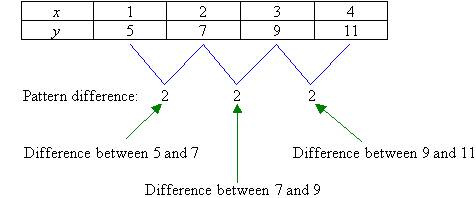We notice that the values of x increase by just one at a time and the difference between the successive values of y is 2.  So, the rule starts off with y = 2x.  Will this give a correct answer from the table?  Let us check.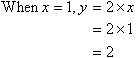The answer is no.  From the table, when x = 1 the value of y should be 5.  How do we change our answer from 2 to 5?  We should add 3.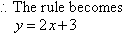Check the rule to see if it is correct: